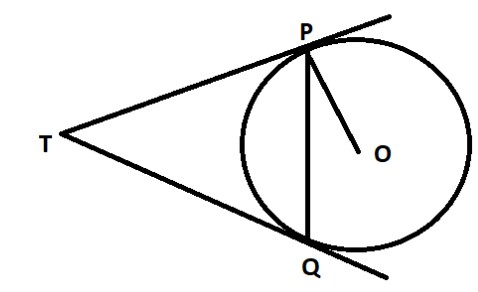QuestionAnswers

# Two tangents TP and TQ are drawn to a circle with centre O from an external point T. Prove that $\angle PTQ = 2\angle OPQ$.Hint: Use the concept that length of tangents drawn from an external point to a circle are equal. So, TP = TQ, which are the tangents drawn from the external point T. Since, TP = TQ, so in triangle TPQ, angles TQP and TPQ will be equal due to the property that opposite angles to equal sides are equal.

Given in the question- A circle with centre O. Two tangents TP and TQ are drawn from external point T.
To prove: $\angle PTQ = 2\angle OPQ$.
Proof:
We know from the theorem that lengths of tangents drawn from an external point to a circle are equal. So, we can say that-
$TP = TQ \\ \Rightarrow \angle TQP = \angle TPQ \to (1) \\$ (Angles opposite to equal sides are equal)
Now, we know TP is tangent and OP is radius.
Therefore, $OP \bot TP$ by the theorem, tangent at any point of a circle is perpendicular to the radius through the point of contact.
So, $\angle OPT = {90^ \circ } \\ \Rightarrow \angle OPQ + \angle TPQ = {90^ \circ } \\ \Rightarrow \angle TPQ = {90^ \circ } - \angle OPQ \to (2) \\$
In $\vartriangle PTQ$
$\angle TPQ + \angle TQP + \angle PTQ = {180^ \circ }$ (Angle sum property of triangle)
$\angle TPQ + \angle TPQ + \angle PTQ = {180^ \circ }(From(1):\angle TQP = \angle TPQ) \\ \Rightarrow 2\angle TPQ + \angle PTQ = {180^ \circ } \\ \Rightarrow 2({90^ \circ } - \angle OPQ) + \angle PTQ = {180^ \circ }(From(2)) \\ \Rightarrow 2({90^ \circ }) - 2\angle OPQ + \angle PTQ = {180^ \circ } \\ \Rightarrow {180^ \circ } - 2\angle OPQ + \angle PTQ = {180^ \circ } \\ \Rightarrow \angle PTQ = 2\angle OPQ \\$
Hence Proved.

Note- Whenever such types of questions appear, then use the basic theorems of mathematics to solve the question. As mentioned in the solution, we have used the theorem that tangents drawn from an external point to a circle are equal and the theorem that the tangent at any point on a circle is perpendicular. Then in triangle PTQ, we have used the angle sum property and by using equation (1) and (2), we have proved the result.
Area of a Sector of a Circle FormulaTo Determine the Mass of Two Different Objects Using a Beam BalanceHow To Make A Buzzer?How to Make A VolcanoHow to Make a Wooden Generator?How to Make a Potato Clock?How to Make a Model of Seasons?How to Find Square Root of a NumberTable 11 to 30CBSE Class 8 Maths Chapter 15 - Introduction to Graphs FormulasImportant Questions for CBSE Class 6 English A Pact with The Sun Chapter 1 - A Tale of Two BirdsImportant Questions for CBSE Class 8 English Honeydew Chapter 7 - A Visit to CambridgeImportant Questions for CBSE Class 11 Maths Chapter 12 - Introduction to Three Dimensional GeometryImportant Questions for CBSE Class 6 English A Pact with The Sun Chapter 9 - What Happened to The ReptilesImportant Questions for CBSE Class 11 Physics Chapter 4 - Motion in a PlaneImportant Questions for CBSE Class 11 Accountancy Chapter 1 - Introduction To AccountingImportant Questions for CBSE Class 11 Business Studies Chapter 7 - Formation of a CompanyImportant Questions for CBSE Class 6 English A Pact with The Sun Chapter 8 - A Pact with the SunImportant Questions for CBSE Class 6 English A Pact with The Sun Chapter 10 - A Strange Wrestling MatchImportant Questions for CBSE Class 11 Physics Chapter 3 - Motion in a Straight LineCBSE Class 10 Hindi A Question Paper with SolutionsCBSE Class 10 Hindi A Question Paper 2020Hindi A Class 10 CBSE Question Paper 2009Hindi A Class 10 CBSE Question Paper 2015Hindi A Class 10 CBSE Question Paper 2016Hindi A Class 10 CBSE Question Paper 2012Hindi A Class 10 CBSE Question Paper 2010Hindi A Class 10 CBSE Question Paper 2007Hindi A Class 10 CBSE Question Paper 2013Hindi A Class 10 CBSE Question Paper 2008RD Sharma Class 11 Maths Solutions Chapter 24 - The CircleNCERT Solutions Class 11 English Woven Words Poem Chapter 11 Ode to a NightingaleRS Aggarwal Class 11 Solutions Chapter-21 CircleRD Sharma Class 11 Solutions Chapter 24 - The Circle (Ex 24.2) Exercise 24.2RD Sharma Class 11 Solutions Chapter 24 - The Circle (Ex 24.1) Exercise 24.1RD Sharma Class 11 Solutions Chapter 24 - The Circle (Ex 24.3) Exercise 24.3RS Aggarwal Class 8 Mathematics Solutions for Chapter-18 Area of a Trapezium and a PolygonNCERT Solutions for Class 10 English First Flight Chapter 1 - A Letter to GodRD Sharma Class 12 Maths Solutions Chapter 16 - Tangents and NormalsTextbooks Solutions for CBSE & ICSE Board of Class 6 to 12 Maths & Science